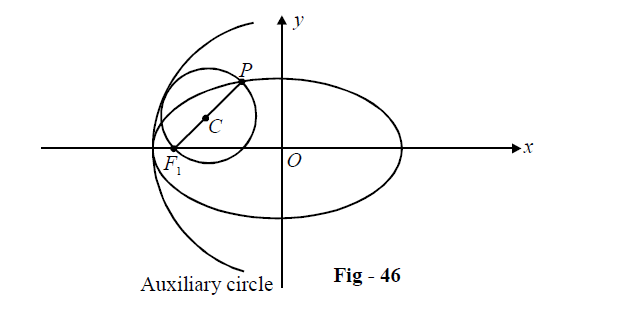# Ellipses Set-5

Go back to  'SOLVED EXAMPLES'

Example – 9

Consider three points on the ellipse \begin{align}\frac{{{x^2}}}{{{a^2}}} + \frac{{{y^2}}}{{{b^2}}} = 1\,,\,\,P({\theta _1}),\,\,Q({\theta _2})\;and\;R({\theta _3}).\end{align} What is the area of $$\Delta PQR\,\,?$$ When is this area maximum?

Solution: The three points have the coordinates

$P \equiv (a\cos {\theta _1},\,\,b\sin {\theta _1});\,\,\,Q \equiv (a\cos {\theta _2},b\sin {\theta _2});\,\,\,R \equiv (a\cos {\theta _3},\,b\sin {\theta _3})$

The area of this triangle, by the determinant formula, is

\Delta = \frac{1}{2}\left| {\begin{align}&{a\cos {\theta _1}}&&{b\sin {\theta _1}}&&1\\&{a\cos {\theta _2}}&&{b\sin {\theta _2}}&&1\\&{a\cos {\theta _3}}&&{b\sin {\theta _3}}&&1\end{align}} \right|\quad\quad\quad...\left( 1 \right)

\begin{align}& = \frac{{ab}}{2}\left\{ {\cos {\theta _1}(\sin {\theta _2} - \sin {\theta _3}) + \sin {\theta _1}(\cos {\theta _3} - \cos {\theta _2}) + \left( {\cos {\theta _2}\sin {\theta _3} - \sin {\theta _2}\cos {\theta _3}} \right)} \right\}\\ &= \frac{{ab}}{2}\left\{ {\sin ({\theta _2} - {\theta _1}) + \sin ({\theta _1} - {\theta _3}) + \sin ({\theta _3} - {\theta _2})} \right\}\\ &= \frac{{ab}}{2}\left\{ {\sin ({\theta _2} - {\theta _1}) + 2\sin \left( {\frac{{{\theta _1} - {\theta _2}}}{2}} \right)\cos \left( {\frac{{{\theta _1} + {\theta _2}}}{2} - {\theta _3}} \right)} \right\}\\ &= ab\sin \left( {\frac{{{\theta _1} - {\theta _2}}}{2}} \right)\left\{ {\cos \left( {{\theta _3} - \frac{{{\theta _1} + {\theta _2}}}{2}} \right) - \cos \left( {\frac{{{\theta _1} - {\theta _2}}}{2}} \right)} \right\}\\ &= 2ab\sin \left( {\frac{{{\theta _1} - {\theta _2}}}{2}} \right)\sin \left( {\frac{{{\theta _2} - {\theta _3}}}{2}} \right)\sin \left( {\frac{{{\theta _3} - {\theta _1}}}{2}} \right)\end{align}

This is the area of the triangle PQR.

To find its maximum value, we use a rather indirect route. Suppose we had to calculate the area $$\Delta '$$ of a triangle inscribed in the circle $${x^2} + {y^2} = {a^2}$$ with the same polar angles as P, Q, R. The only difference between $$\Delta '\;and\;\Delta$$ will be that in the determinant expression for $$\Delta$$ in (1), we will have all ‘a’ instead of ‘b’ in the terms of the second column.

This means that $$\Delta \;and\;\Delta '$$ will always be in a constant ratio:

$\frac{\Delta }{{\Delta '}} = \frac{b}{a}$

Thus, the maximum for $$\Delta$$ will be achieved in the same configuration as the one in which the maximum of $$\Delta '$$ be achieved!

Since the area of a triangle inscribed in a circle has the maximum value when that triangle is equilateral (this should be intuitively obvious but can also be easily proved), $$\Delta '$$ and hence $$\Delta$$ will

be maximum when

$\left| {{\theta _1} - {\theta _2}} \right| = \left| {{\theta _2} - {\theta _3}} \right| = \left| {{\theta _3} - {\theta _1}} \right| = \frac{{2\pi }}{3}$

Thus, the three eccentric angels must be equally spaced apart at $$\frac{{2\pi }}{3}.$$

Example – 10

Prove that the circle on any focal distance as diameter touches the auxiliary circle of the ellipse.

Solution: Let $$P(\theta )$$ be an arbitrary point on the ellipse $$\frac{{{x^2}}}{{{a^2}}} + \frac{{{y^2}}}{{{b^2}}} = 1$$ and let F1 be one of its foci.The radius of the auxiliary is a. The circle on PF1 as diameter will touch the auxiliary circle (internally) if:

$OC + ({\rm{radius\; of\; this\; circle}}) = a$

C, being the mid-point of PF1, has the coordinates

$C \equiv \left( {\frac{{a\cos \theta - ae}}{2},\,\,\frac{{b\sin \theta }}{2}} \right)$

Thus,

\begin{align}&OC = \sqrt {{{\left( {\frac{{a\cos \theta - ae}}{2}} \right)}^2} + {{\left( {\frac{{b\sin \theta }}{2}} \right)}^2}} \\&\quad~{\rm{ }} = \frac{1}{2}\sqrt {{a^2}{{\cos }^2}\theta + {b^2}{{\sin }^2}\theta + {a^2}{e^2} - 2{a^2}e\cos \theta } \\&\quad~{\rm{ }} = \frac{a}{2}\sqrt {{{\cos }^2}\theta + (1 - {e^2}){{\sin }^2}\theta + {e^2} - 2e\cos \theta } \\&\quad{\rm{ }} = \frac{a}{2}\sqrt {1 + {e^2}{{\cos }^2}\theta - 2e\cos \theta } = \frac{a}{2}(1 - e\cos \theta )\end{align}

Also, the radius of the inner circle is

\begin{align}&C{F_1} \equiv \sqrt {{{\left( {\frac{{a\cos \theta - ae}}{2} + ae} \right)}^2} + {{\left( {\frac{{b\sin \theta }}{2}} \right)}^2}} \\&\quad~~~{\rm{ }} = \frac{a}{2}\sqrt {{{\cos }^2}\theta + (1 - {e^2}){{\sin }^2}\theta + {e^2} + 2\cos \theta } = \frac{a}{2}(1 + e\cos \theta )\end{align}

This gives

$$OC + C{F_1} = a$$ which proves the stated assertion.

Learn from the best math teachers and top your exams

• Live one on one classroom and doubt clearing
• Practice worksheets in and after class for conceptual clarity
• Personalized curriculum to keep up with school